# How to Calculate and Solve for Flexural Strength for Rectangular Cross-section in Defects | Ceramics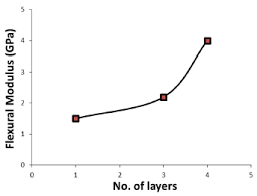The image above represents flexural strength for rectangular cross-section in defects.

To compute for flexural strength for rectangular cross-section in defect, four essential parameters are needed and these parameters are Load at fracture (Ff), Length of the cross-section (b), Width of the cross-section (d) and Distance between support points (L).

The formula for calculating flexural strength for rectangular cross-section in defects:

σfs = 3FfL / 2bd²

Where:

σfs = Flexural Strength
L = Distance between Support Points
b = Length of Cross-section
d = Width of Cross-section

Let’s solve an example;
Find the flexural strength when the load at fracture is 10, the length of cross-section is 7, width of cross-section is 9 and the distance between support points is 21.

This implies that;

Ff = Load at Fracture = 10
L = Distance between Support Points = 21
b = Length of Cross-section = 7
d = Width of Cross-section = 9

σfs = 3FfL / 2bd²
σfr = 3(10)(21) / 2(7)(9)²
σfr = (630) / 2(7)(81)
σfr = (630) / (1134)
σfr = 0.55

Therefore, the flexural strength for rectangular cross-section in defect is 0.55 Pa.

Calculating the Distance Between Support Points when the Flexural Strength for Rectangular Cross-Section in Defects, Load at Fracture, length of the cross-section and width of cross-section is Given.

L = σfs x 2bd2 / 3Ff

Where;

L = Distance between Support Points
σfs = Flexural Strength
b = Length of Cross-section
d = Width of Cross-section

Let’s solve an example;
Find the distance between support points when the flexural strength is 20, the load at fracture is 12, the length of cross-section is 7 and the width of cross-section is 9.

This implies that;

σfs = Flexural Strength = 20
Ff = Load at Fracture = 12
b = Length of Cross-section = 7
d = Width of Cross-section = 9

L = σfs x 2bd2 / 3Ff
L = 20 x 2 x 7 x 92 / 3 x 12
L = 280 x 81 / 36
L = 22680 / 36
L = 630

Therefore, the distance between support points is 630.

Calculating the Load at Fracture when the Flexural Strength for Rectangular Cross-Section in Defects, Distance Between Support Points, Length of Cross-section and Width of Cross-section is Given.

Ff = σfs x 2bd2 / 3L

Where;

σfs = Flexural Strength
L = Distance between Support Points
b = Length of Cross-section
d = Width of Cross-section

Let’s solve an example;
Find the load at fracture when the flexural strength is 40, the distance between support points is 22, the length of cross-section is 15 and the width of cross-section is 12.

This implies that;

σfs = Flexural Strength = 40
L = Distance between Support Points = 22
b = Length of Cross-section = 15
d = Width of Cross-section = 12

Ff = σfs x 2bd2 / 3L
Ff = 40 x 2 x 15 x 122 / 3 x 22
Ff = 1200 x 144 / 66
Ff = 172800 / 66
Ff = 2618.1

Therefore, the load at fracture is 2618.1.

Calculating the Length of Cross-Section when the Flexural Strength for Rectangular Cross-Section in Defects, Distance Between Support Points, Load at Fracture and Width of Cross-section is Given.

b = 3Ff / σfs x 1 / 2d2

Where;

b = Length of Cross-section
σfs = Flexural Strength
L = Distance between Support Points
d = Width of Cross-section

Let’s solve an example;
Find the lenght of cross-section when the flexural strength is 24, the load at fracture is 10, the distance between support points is 8 and the width of cross-section is 3.

This implies that;

σfs = Flexural Strength = 24
Ff = Load at Fracture = 10
L = Distance between Support Points = 8
d = Width of Cross-section = 3

b = 3Ff / σfs x 1 / 2d2
b = 3 x 10 / 24 x 1 / 2 x 32
b = 30 / 24 x 1 / 2 x 9
b = 1.25 x 1 / 18
b = 1.25 x 0.05
b = 0.0625

Therefore, the length of cross-section is 0.0625.

Calculating the Width of Cross-Section when the Flexural Strength for Rectangular Cross-Section in Defects, Distance Between Support Points, Load at Fracture and Length of Cross-Section is Given.

d = √(3Ff / σfs x 1 / 2b)

Where;

d = Width of Cross-section
σfs = Flexural Strength
L = Distance between Support Points
b = Length of Cross-section

Let’s solve an example;
Find the width of cross-section when the flexural strength is 14, load at fracture is 6, distance between support points is 4 and length of cross-section is 2.

This implies that;

σfs = Flexural Strength = 14
Ff = Load at Fracture = 6
L = Distance between Support Points = 4
b = Length of Cross-section = 2

d = √(3Ff / σfs x 1 / 2b)
d = √(3 x 6 / 14 x 1 / 2 x 2)
d = √(18 / 14 x 1 / 4)
d = √(1.28 x 0.25)
d = √(0.32)
d = 0.56

Therefore, the width of cross-section is 0.56.

Nickzom Calculator – The Calculator Encyclopedia is capable of calculating the flexural strength for rectangular cross-section in defects.

To get the answer and workings of the flexural strength for rectangular cross-section in defects using the Nickzom Calculator – The Calculator Encyclopedia. First, you need to obtain the app.

You can get this app via any of these means:

To get access to the professional version via web, you need to register and subscribe for NGN 1,500 per annum to have utter access to all functionalities.
You can also try the demo version via https://www.nickzom.org/calculator

Apple (Paid) – https://itunes.apple.com/us/app/nickzom-calculator/id1331162702?mt=8
Once, you have obtained the calculator encyclopedia app, proceed to the Calculator Map, then click on Materials & Metallurgical under Engineering.Now, Click on Ceramics under Material & MetallurgicalNow, Click on Flexural Strength for Rectangular Cross-Section in Defects under CeramicsThe screenshot below displays the page or activity to enter your values, to get the answer for the flexural strength for rectangular cross-section in defects  according to the respective parameters which are the Load at fracture (Ff), Length of the cross-section (b), Width of the cross-section (d) and Distance between support points (L).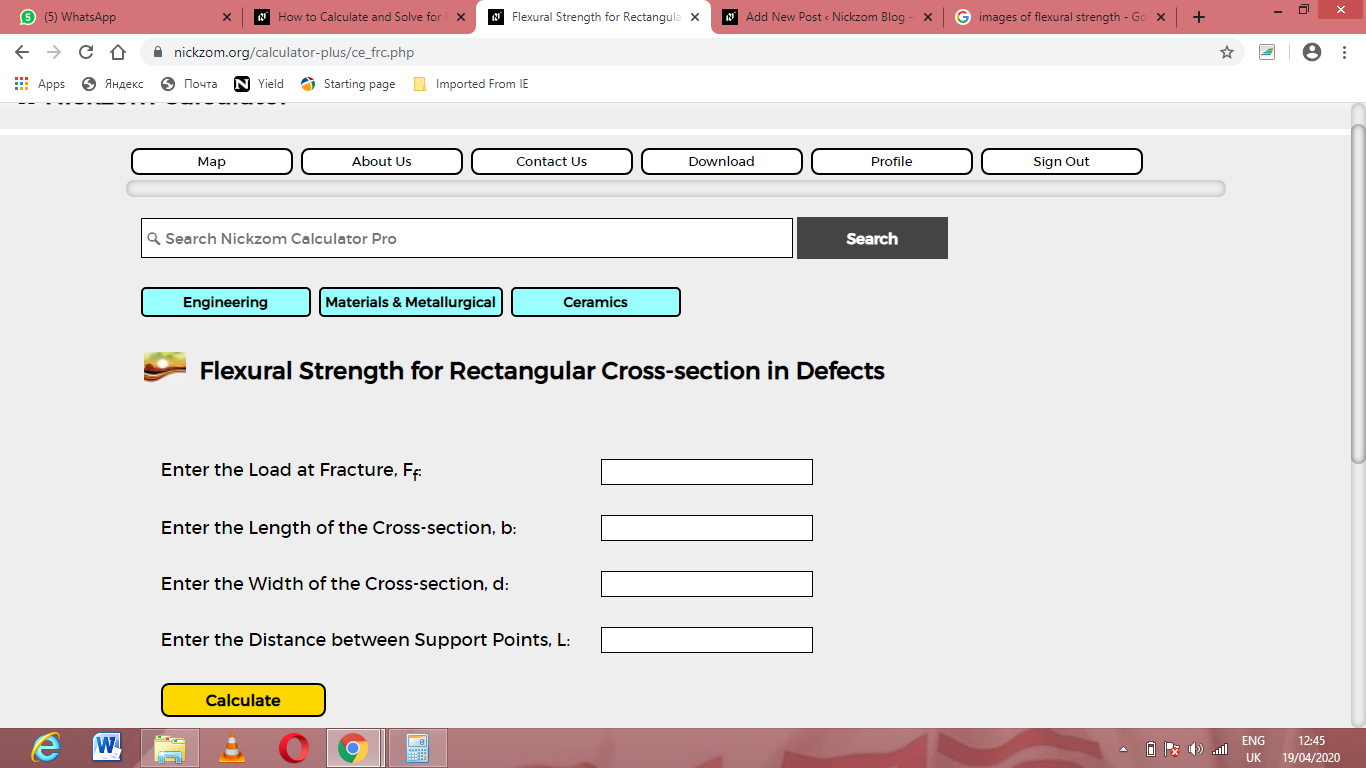Now, enter the values appropriately and accordingly for the parameters as required by the Load at fracture (Ff) is 10, Length of the cross-section (b) is 7, Width of the cross-section (d) is 9 and Distance between support points (L) is 21.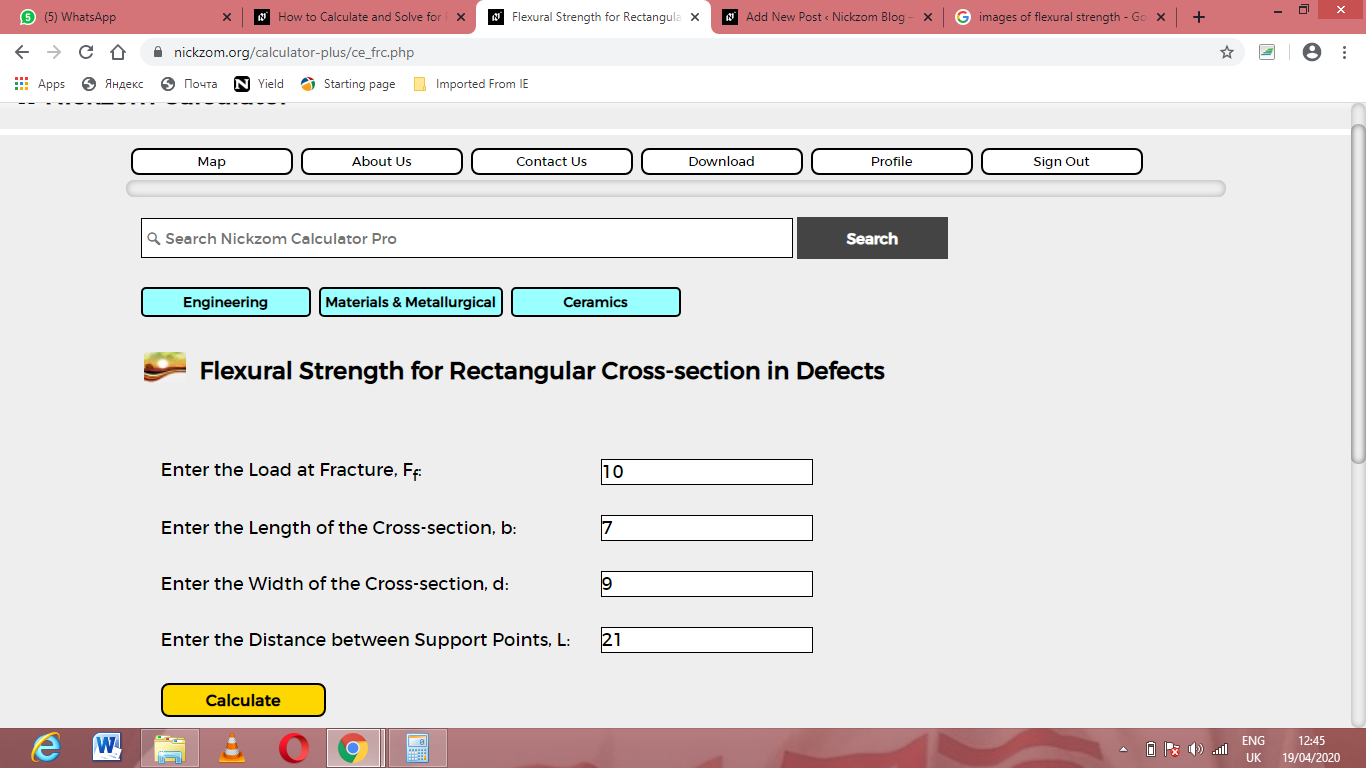Finally, Click on Calculate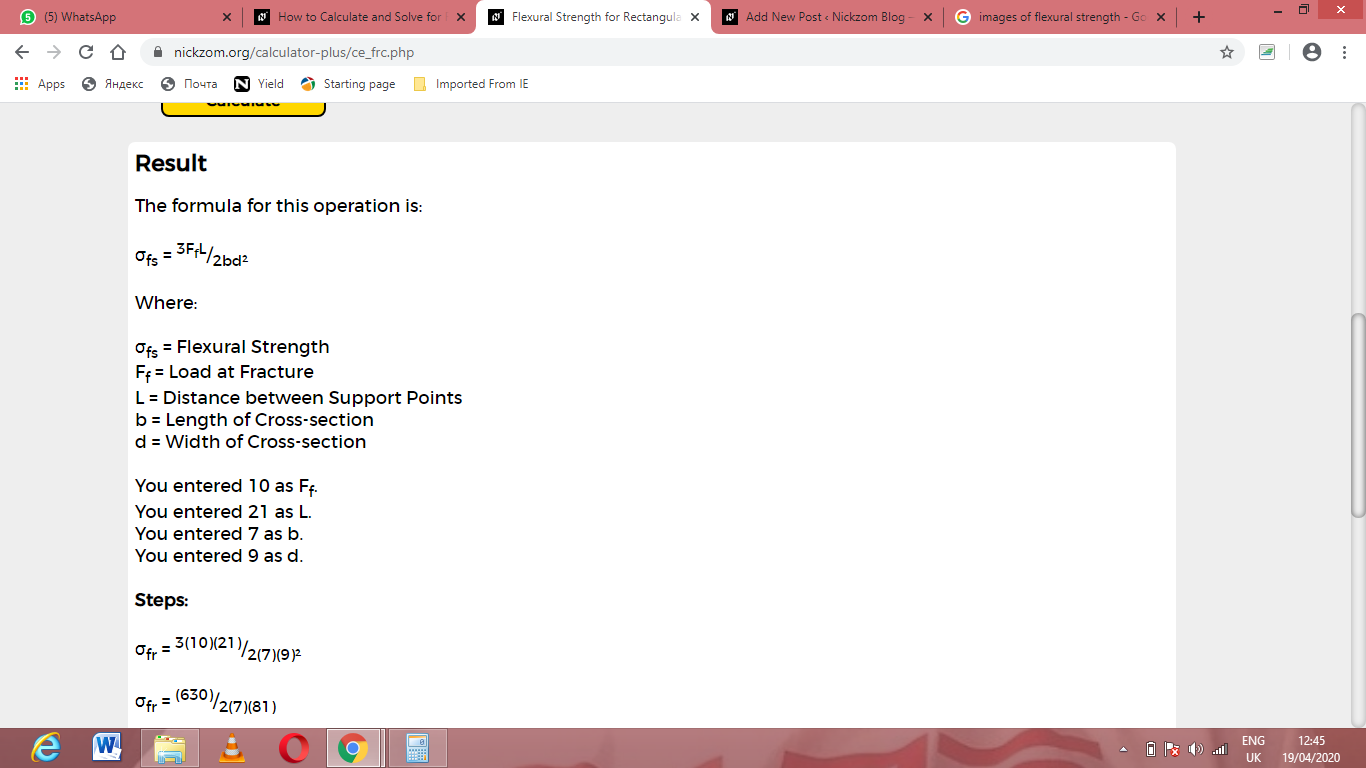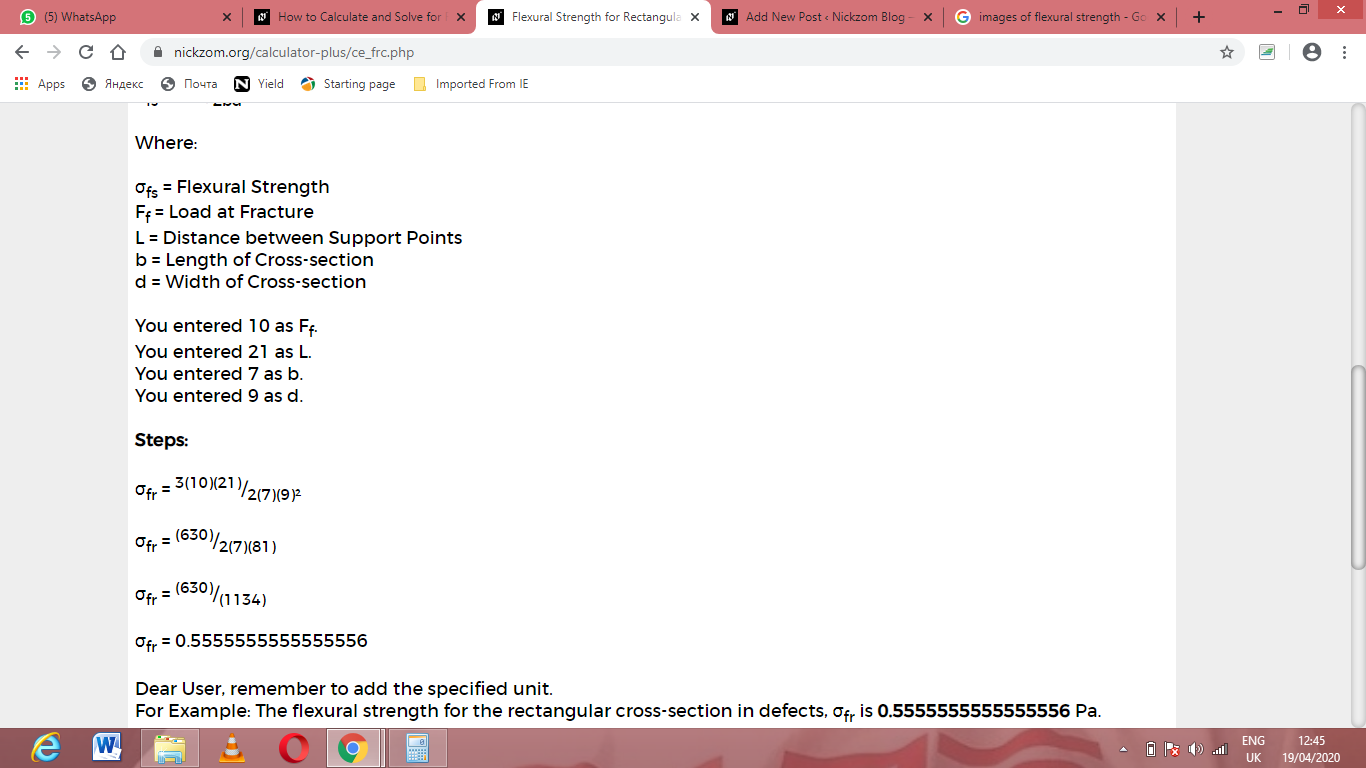As you can see from the screenshot above, Nickzom Calculator– The Calculator Encyclopedia solves for the flexural strength for rectangular cross-section in defects and presents the formula, workings and steps too.